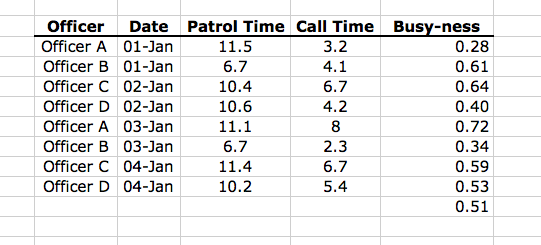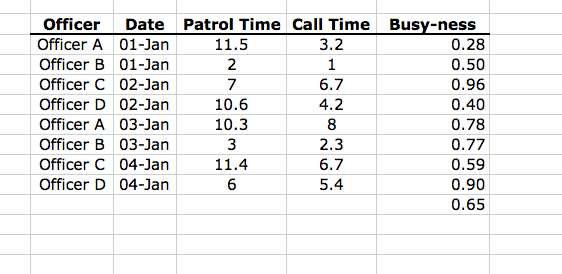### Being Careful with Averages

In crime analysis we are used to calculating averages and when I say average I mean the “mean”—e.g. add up ten numbers and divide by ten—that kind of average. But in crime analysis we are also frequently interested in examining rates or ratios. For example: how many hours of patrol time are spent responding to calls. When we combine the two there is the potential to be tripped up since, if we are talking ratios, we need to be very careful about how we calculate an average. Depending on the approach we take we’ll get different numbers.

An example will help illustrate the problem.

Let’s say that your CAD system keeps track of how many hours an officer spends on patrol and, of that time, how much of it is spent responding to calls. The following table illustrates a small sample of this kind of data.From the data in the table I calculated a ‘busy-ness’ ratio for each officer; this is Call Time divided by Patrol Time. From those values I in turn calculated the average Busy-ness, which is 0.51, or 51% busy. But wait, let’s add up all the Patrol Time (78.6 hours) and all the Call Time (40.6 hours) and find the ratio. (40.6/78.6)=0.52 or 52% busy. These numbers are different because of the mathematical principle that says that the ratio of averages (0.52) does not necessarily equal the average of ratios (0.51). But which one is ‘correct’? Well, they both are, and the one you go with all depends on what question you are trying to answer.

For the ratio of averages (0.52) we are asking a question about the entire police service: “how much patrol time did we spend on calls?” For this question 52% is the correct answer because it takes into account the total time spent on patrol and the total time spent on calls. If an officer spends 3 hours on patrol or 12 hours, all of that is taken into account in the final number.

The average of ratios (0.51) is trickier as it answers a question about officers going on patrol: “how much of their patrol can an officer expect to spend on calls?” The difference here arises because the average of ratios treats all values contributing to the mean as having equal weight and this is a fine assumption when the question is focused on how the averages behave. In other words, the length of the shift doesn’t matter, only how much of the shift was spent on calls.

But why am I telling you this since the difference seems to be pretty small? It’s because there are situations where the difference can be significant. For example, look at the modified table below where by adjusting the patrol times I was able to increase the difference between the averages to 4%. Making a 4% mistake on something like officer busy-ness can be the difference between hiring more officers and not. That’s not a mistake I would want hanging around my neck.The take away from this post is to be mindful when taking averages because so many of the numbers crime analysts look at are ratios. Whether we’re looking at officer busy-ness or per capita car thefts or tickets issued per traffic blitz it is important to understand the question that the average is supposed to answer.

Bonus commentary for pedants
But wait, you might be saying, how is the 0.52 number a ratio of averages? No average was even calculated; we just summed the numbers and divided the Patrol Time by the Call Time. That’s a ratio of sums. Yes, that’s true, but if you had wanted to be a real stickler you could have found the average Patrol Time (78.6/8 = 9.825) and the Call Time (40.6/8 = 5.075) and divided those numbers and arrived at the same answer (5.075/9.825) = 0.52. It works this way because the divisor in both the averages is the same (it’s 8 representing the 8 shifts included in the calculation) and therefore it cancels out when the ratio is taken.